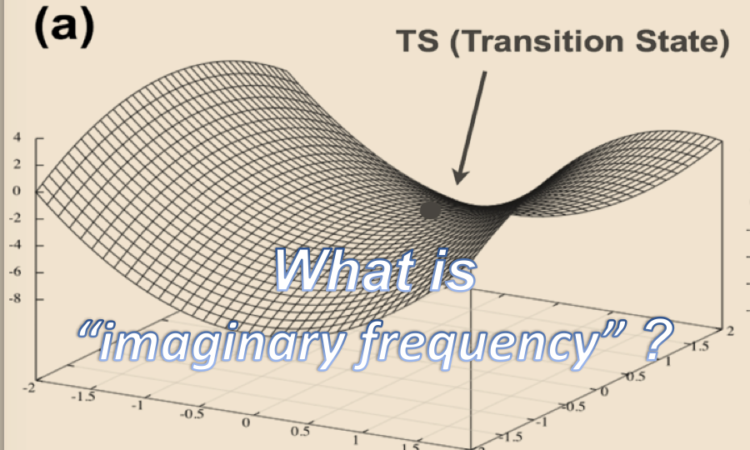# Transition State? Imaginary Frequency?

Computational chemistry can clarify transition state structures, reaction pathways, activation energies and so on…

Nowadays, since the gaussian interface is very user-friendly, anyone can conduct the transition state optimization and IRC calculations. Many people know that transition state has single imaginary frequency, however, only few of them know what it does mean…

In this article, we will explain what the imaginary frequency is, and what the transition state is.

Most people might be familiar with 2 dimensional energy diagram Fig 1 (a), and we guess that you might have learned the local maxima is the transition state. However, real Potential Energy Surface (PES) has 3N-6 dimension.

For simplicity, we use 3-D dimensional PES Fig1(b).  In this figure, transition state is on the SADDLE POINT, which is the local maxima from one direction and also local minima from other directions.Fig 1. 2D-energy diagram and 3D-energy diagram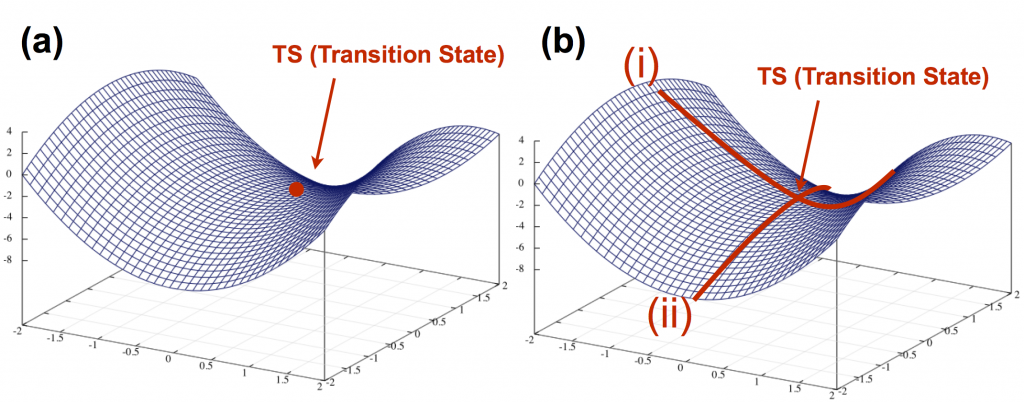Fig2. (a): SADDLE POINT (b): local minima from direction (i) and also local maxima from direction (ii)

In the transition state calculation, you need to specify the keyword opt=ts in Gaussian, or SADDLE in GRRM. Even if these calculations are normally terminated, you can’t say that this structure is the transition state. First you need to check the Vibration mode. Second you need perform IRC calculation to confirm if it’s the transition state structure of the reaction you pursued.

## Transition state structure is the structure that has single imaginary frequency.

### What is imginary frequency ?

The equation of the harmonic oscillator is almost the same equation as spring equation of the law of hook of classical mechanics. If the spring is shortened or extended, the restoring force trying to return to the original works. Likewise, when the coordinates move a little from the local minima, the restoring force which normally tries to return to the minima works (Fig. 3).Fig 3.  Energy diagrams of local minima

However, in the transition state, it proceeds in the direction toward the intermediate structure, and the restoring force doesn’t work.Fig 4.  Energy diagrams of local maxima

Equation of reference vibration can be expressed by the following equation.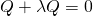This equation is the same as the vibration of the spring, and λ corresponds to the restoring force of the spring. It can be expressed by the following expression.In the case <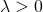,>In the case <,>The frequency v can be expressed by the following equation.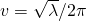That is, it can be summarized as follows.

When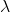is positive,is real number ： The restoring force works, it becomes harmonic vibration.

Whenis negative,is imaginary number ： No restoring force.

As shown here, it is possible to see the shape of PES by performing frequency analysis. In other words, PES is convex upward in the case of imaginary vibration, and PES is convex downward in the case of real vibration.

By the way, the point which has two or more imaginary vibrations is called the high order saddle point (maximum point), not the transition state.

### IRC calculation

Even if you confirm that there is single imaginary frequency, it is still unclear whether it is the desired transition state. Therefore, it is necessary to carry out the IRC calculation. IRC stands for Intrinsic Reaction Coordinate. In the IRC calculation, the transition state structure is moved little by little along the direction of the imaginary frequency. This is the operation to move a structure from the state in Fig. 4 (a) to the state in Fig. 4 (b). For more intuitive explanation, it can be expressed as calculating how far a ball rolls from the transition state on the PES. During this process, the ball constantly reaches the local minima through the minimum energy path (Variational principle).

In Gaussian, after reaching the structure shown in Fig. 4 (b) by IRC calculation, you can obtain the structure of the intermediate by optimization calculation. A common mistake is to calculate transition state structure and intermediate structure separately without performing IRC calculation. There are many mistakes in people who do not specialize in calculations.

We will explain the IRC calculation in another article. We know that the concept of imaginary frequency is hard to understand at first. We think that it will lead to a deep understanding that being able to explain what is meant by each figure, mathematical expression, actual molecular structure and 2D & 3D PES.

#### Related Artcles

This site uses Akismet to reduce spam. Learn how your comment data is processed.